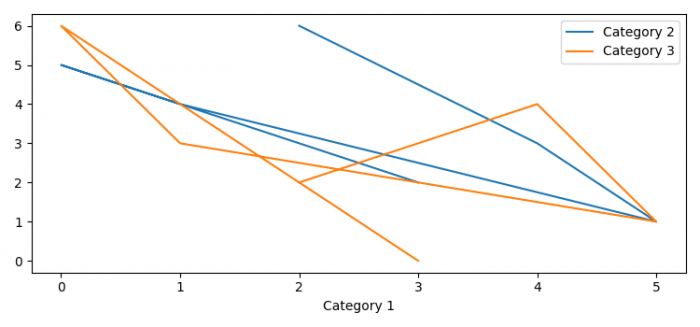# How to plot multiple Pandas columns on the Y-axis of a line graph (Matplotlib)?

To plot multiple Pandas columns on the Y-axis of a line graph, we can set the index using set_index() method.

## Steps

• Set the figure size and adjust the padding between and around the subplots.
• Create a dataframe with Category 1, Category 2, and Category 3 columns.
• Use set_index() method to set the DataFrame index using existing columns.
• To display the figure, use show() method.

## Example

import pandas as pd
from matplotlib import pyplot as plt

plt.rcParams["figure.figsize"] = [7.50, 3.50]
plt.rcParams["figure.autolayout"] = True
df = pd.DataFrame({'Category 1': [2, 4, 5, 1, 0, 3],
'Category 2': [6, 3, 1, 4, 5, 2],
'Category 3': [2, 4, 1, 3, 6, 0]})
df.set_index('Category 1').plot()
plt.show()

## Output